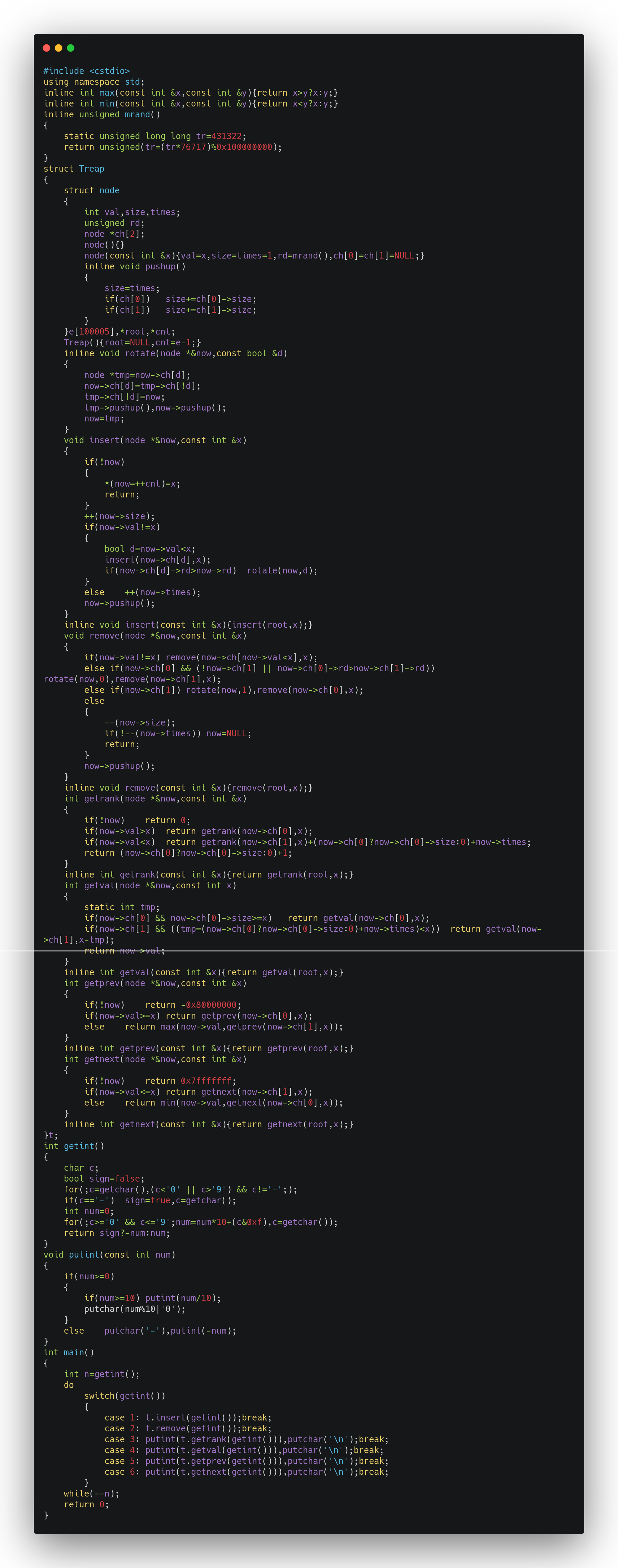# 一、操作&实现

## 1. 存储

struct Treap
{
struct node
{
int val,size,times;
unsigned rd;
node *ch;
}e,*root,*cnt;
};


• Treap：表示 Treap
• node：表示节点。
• val：表示该节点所储存的数值。
• size：表示以该节点为根的子树的大小（节点总数）。
• times：表示该节点的数值的存在数量。
• rd：随机优先值。
• ch：指向子节点的指针。
• ch：指向左儿子的指针。
• ch：指向右儿子的指针。
• e：储存所有节点。
• root：指向根节点的指针。
• cnt：指向最后一个插入的节点的指针。

## 2. 操作

### 2-0 node 的操作

#### 2-0-1 构造函数

node(){}
// 插入节点时用
node(const int &x){val=x,size=times=1,rd=rand(),ch=ch=NULL;}

##### 优化

C++ 自带的 rand 函数速度较慢，我们可以自己写一个 mrand 函数来取代：

inline unsigned mrand()
{
static unsigned long long tr=431322;
return unsigned(tr=(tr*76717)%0x100000000);
}


node(){}
// 插入节点时用
node(const int &x){val=x,size=times=1,rd=mrand(),ch=ch=NULL;}


#### 2-0-2 更新节点信息

inline void pushup()
{
size=times;
if(ch)	size+=ch->size;
if(ch)	size+=ch->size;
}


struct node
{
int val,size,times;
unsigned rd;
node *ch;
node(){}
node(const int &x){val=x,size=times=1,rd=mrand(),ch=ch=NULL;}
inline void pushup()
{
size=times;
if(ch)	size+=ch->size;
if(ch)	size+=ch->size;
}
};


// now 表示所操作子树的根节点指针引用，indent 表示当前缩进长度
void output(node *&now,const int &indent)
{
putchar('>'),putchar(' ');
for(int i=0;i<indent;++i)	putchar('|'),putchar(' ');
if(!now)
{
puts("NULL");
return;
}
// 输出当前节点的详细信息，可以更改
printf("%ld:%d %d,%d %u\n",now-e,now->val,now->size,now->times,now->rd);
output(now->ch,indent+1);
output(now->ch,indent+1);
}
// 初始函数（方便调用）
inline void output(){output(root,0);}


### 2-1 旋转// now 表示所操作子树的根节点指针引用，d 表示旋转方向（0 表示右旋，1 表示左旋）
// 这里 now 为引用类型，便于更改。
inline void rotate(node *&now,const bool &d)
{
node *tmp=now->ch[d];			// tmp 指向将成为新的根节点的节点
now->ch[d]=tmp->ch[!d];
tmp->ch[!d]=now;
tmp->pushup(),now->pushup();	// 更新节点信息
now=tmp;						// tmp 指向的节点成为新的根节点
}


### 2-2 插入值为 $x$ 的节点

Treap 的插入其实就是在二叉排序树的插入的基础上通过旋转保证 Treap 堆的性质。

// now 表示指向当前节点的指针的引用，x 表示要插入的值
// 这里 now 为引用类型，便于更改。
void insert(node *&now,const int &x)
{
// 如果为空指针
if(!now)
{
*(now=++cnt)=x;		// 插入新节点
return;
}
++(now->size);			// 因为新节点在以 now 指向的节点为根节点的子树内，所以当前子树的节点数+1
// 如果当前节点的数值不等于 x
if(now->val!=x)
{
bool d=now->val<x;	// d 为插入的方向（0 为左，1 为右）
insert(now->ch[d],x);
// 如果当前节点的子节点的优先值大于当前节点的优先值（即不符合堆的性质）
if(now->ch[d]->rd>now->rd)	rotate(now,d);	// 旋转以维护堆的性质
}
// 否则说明当前节点的数值等于 x
else	++(now->times);	// 当前节点的数值的存在数量+1
now->pushup();			// 更新当前节点（之前我没有加上，导致我调了好久的 BUG）
}
// 初始函数（方便调用）
inline void insert(const int &x){insert(root,x);}


### 2-3 删除值为 $x$ 的节点

// now 表示指向当前节点的指针的引用，x 表示要删除的值
// 这里 now 为引用类型，便于更改。
void remove(node *&now,const int &x)
{
// 如果当前节点不为要删除的节点
if(now->val!=x)	remove(now->ch[now->val<x],x);	// 继续向下寻找
// 否则说明要删除当前节点
// 如果当前节点有左儿子并且左儿子的优先值大于右儿子的优先值
// 则右旋使左儿子代替被删除的节点的位置
else if(now->ch && (!now->ch || now->ch->rd>now->ch->rd))	rotate(now,0),remove(now->ch,x);
// 否则如果当前节点有右儿子
// 则左旋使右儿子代替被删除的节点的位置
else if(now->ch)	rotate(now,1),remove(now->ch,x);
// 否则说明当前节点没有子节点
else
{
--(now->size);
// 如果该节点的存在数量清零了
if(!--(now->times))	now=NULL;	// 直接删除
return;
}
now->pushup();// 更新当前节点
}
// 初始函数（方便调用）
inline void remove(const int &x){remove(root,x);}


### 2-4 查询数值为 $x$ 的节点的排名

// now 表示指向当前节点的指针的引用，x 表示要查询的值
int getrank(node *&now,const int &x)
{
// 如果为空指针，说明改数不存在于树中
if(!now)	return 0;							// 直接返回 0
// 如果当前节点的值大于 x
if(now->val>x)	return getrank(now->ch,x);	// 继续搜索左子树
// 如果当前节点的值小于 x
// 继续搜索右子树，返回值增加左子树节点数+当前节点存在数量
if(now->val<x)	return getrank(now->ch,x)+(now->ch?now->ch->size:0)+now->times;
// 否则说明当前节点的值等于 x
return (now->ch?now->ch->size:0)+1;		// 返回左子树节点数+1
}
// 初始函数（方便调用）
inline int getrank(const int &x){return getrank(root,x);}


### 2-5 查询排名为 $x$ 的节点的数值

// now 表示指向当前节点的指针的引用，x 表示要查询的排名
int getval(node *&now,const int x)
{
static int tmp;	// 临时变量，用于储存左子树的节点数+当前节点存在数量，为了节省空间就使用静态变量了
// 如果当前节点有左儿子且左子树的节点数不小于 x
// 继续搜索左子树
if(now->ch && now->ch->size>=x)	return getval(now->ch,x);
// 否则如果当前节点有右儿子且左子树的节点数+当前节点存在数量小于 x
// 继续搜索右子树中排名为 x-tmp 的节点
if(now->ch && ((tmp=(now->ch?now->ch->size:0)+now->times)<x))	return getval(now->ch,x-tmp);
// 否则说明当前节点即要查询的节点
return now->val;// 返回当前节点的数值
}
// 初始函数（方便调用）
inline int getval(const int &x){return getval(root,x);}


### 2-6 查询数值为 $x$ 的节点的前驱

// now 表示指向当前节点的指针的引用，x 表示要查询的数值
int getprev(node *&now,const int &x)
{
// 如果为空指针
if(!now)	return -0x80000000;						// 返回负无穷
// 如果当前节点的数值不小于 x
// 说明前驱在左子树内
if(now->val>=x)	return getprev(now->ch,x);		// 搜索左子树
// 否则说明前驱为当前节点或在右子树内
else	return max(now->val,getprev(now->ch,x));	// 搜索右子树
}
// 初始函数（方便调用）
inline int getprev(const int &x){return getprev(root,x);}


### 2-7 查询数值为 $x$ 的节点的后继

// now 表示指向当前节点的指针的引用，x 表示要查询的数值
int getnext(node *&now,const int &x)
{
// 如果为空指针
if(!now)	return 0x7fffffff;						// 返回负无穷
// 如果当前节点的数值不大于 x
// 说明后继在左子树内
if(now->val<=x)	return getnext(now->ch,x);		// 搜索左子树
// 否则说明后继为当前节点或在右子树内
else	return min(now->val,getnext(now->ch,x));	// 搜索右子树
}
// 初始函数（方便调用）
inline int getnext(const int &x){return getnext(root,x);}


# 二、例题

## 1. 洛谷 P3369 【模板】普通平衡树posted @ 2020-03-17 19:31  Createsj  阅读(312)  评论(0编辑  收藏  举报